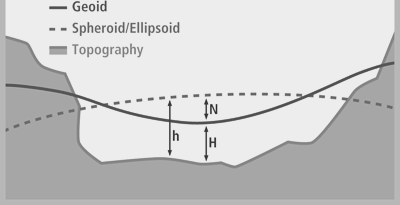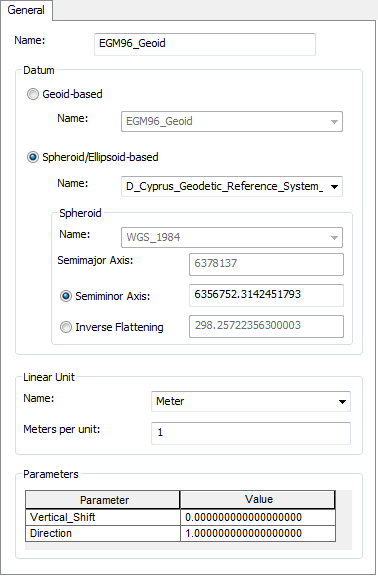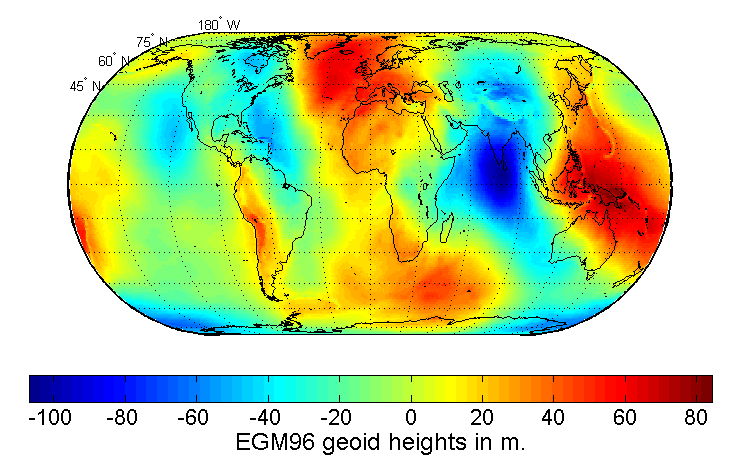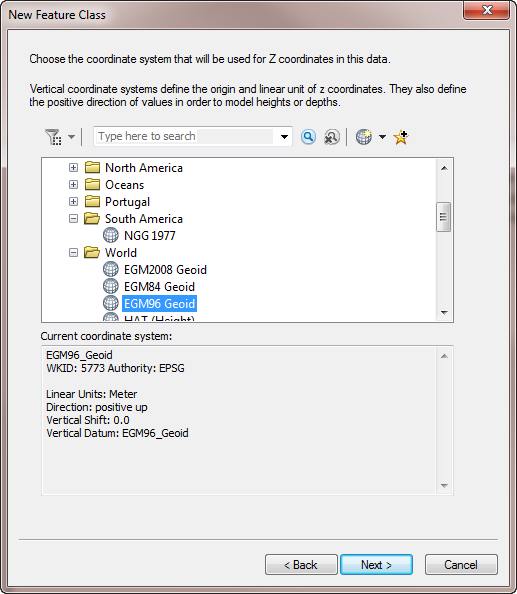# Coordinate Reference Systems Blog

cancel
Showing results for
Did you mean:

(28 Posts)

## The Vertical Datum: Or is that Mean Sea level?

Regular Contributor

What is a Vertical Datum?

The Geodetic Glossary (2009) defines height as ‘distance, measured along a perpendicular, between a point and a reference surface’. This definition is concise and direct but leaves a vagueness concerning the reference surface. This reference surface is the Vertical Datum. A datum is required to reach accurate and reliable measures of height above a surface.

The EPSG dataset currently lists 128 vertical datums worldwide. There are various types of height including:

• Ellipsoidal (The difference between the topographic elevation and the ellipsoid)
• Orthometric (The difference between the topographic elevation and the geoid)
• Geoid (The difference between the ellipsoidal height and the geoid surface Note this can be negative)

Heights are categorized into two types, those with reference to the Earth's gravity field and those defined on geometry alone. It is important to note that the two categories of height are not directly interchangeable as they are completely different. Where the gravity field heights are based on the geoid that is defined by a eqipotential surface‌ compared to, for example, the height of a tree using right angle geometry where ground level is defined as 0 height.Figure 1 Difference between the Geoid and Spheroid/Ellipsoid based surfaces and real topography (adapted from ESRI 2012) where h = Ellipsoidal height, N = Orthomatric height and H = Geoid height.

In my experience height is colloquially referred to as X units above or below Mean Seal Level (MSL). Sea level comes from the Earth' gravity field, therefore gravity is studied to understand height.

So, what is mean sea level?

In its simplest form MSL is the average location for the surface of the ocean measured over time to minimise random and periodic variation, such as tides and storm surges. The period of time to measure these variations in the ocean surface was set at 19 years by the U.S. National Ocean Surface, these measurements can be brought together to form a tidal datum.

However in this form MSL is not adequate as a global vertical datum as this mean calculation only exists at the station of measurement and its immediate vicinity, plus the ocean has a dynamic topography that is nicely summarised in this MinutePhysics video.

Why is a Vertical Datum important?

I work in the Oil and Gas industry and the application of a correct vertical datum is important in exploration, when defining a height or depth to a geological surface, and also when designing and implementing infrastructure projects such as pipelines, ports and other coastal infrastructure as well as when considering the environmental impacts that spills and other developments may have on coastlines and rivers. For example misunderstanding the vertical datum can impact planning for a storm surge that may have been measured on a different surface when compared to the height of a constructed LNG plant.

In essence there are 2 types of datums used in Vertical Coordinate Reference Systems:

## Geoid-based versus Spheroid/Ellipsoid-based

The Geoid is a reference surface of the Earth and is based on the relationship between gravitational force and gravitational potential that produces a surface where all points are perpendicular to the force of gravity. Meyer (2010) provides an extensive review on the Geoid and the physics behind its construction. This surface is considered the proper surface to create geodetic heights and will nominally correspond to mean sea-level (see the previous video).

Recently there has been a move to see if data from the Gravity field and steady-state Ocean Circulation Explorer (GOCE) ESA project is able to be used with calculating heights and connecting height systems (see here). This will create a very high resolution Geoid for calculating heights across the globe.

Some examples of Geoid surfaces include:

• EGM2008 Geoid
• EGM84 Geoid
• EGM96 Geoid

Spheroid/Ellipsoid-based surfaces use geometric calculations to define  the local datum and a spheroid.

It is important to note that it is impossible to transform between the geoid and Spheroid/Ellipsoid-based datums. This is because there are no common elements between the two reference systems and  no site-specific gravity measurements in a spheroid/ellipsoid system so no mathematical transformation can be applied.

Some examples of Spheroidal/Ellipsoid-based surfaces found in ArcGIS 10.2 include:

• D_Cyprus_Geodetic_Reference_System_1993
• D_Kuwait_Oil_Company
• D_St_Lucia_1955Figure 2 Example of the difference parts that make up a Vertical Datum as presented in ArcGIS.

The Geoid has a more complex surface than a Spheroid/Ellipsoid-based surface where the Spheroid/Ellipsoid-based surface shows a constant rate between chosen parameters of calculation whilst the geoid is ‘bumpy’ indicating the changing gravity measurements that represent the ‘real’ topography of the Earth (Figure 3).Figure 3 The EGM96 Geoid. Taken from Wikipedia.

How do you set a vertical coordinate system in ArcGIS

When creating a new feature class tick the “Coordinates include Z values” option. This will enable the 3D measure of the feature class (Figure 4).Figure 4 New Feature Class: Select Z values to enable 3D use

Click ‘Next’ to select the Geographic/Projected Coordinate System you want to use and then select the Vertical Coordinate System you would like to use.Figure 5. Choose the correct vertical coordinate system for you area of interest. Note that local Vertical CRS have been calculated from locally derived measurements and that MSL (Depth) and MSL (Height) use the EGM96 Geoid.

## Summary and How to transform between Vertical Coordinate Reference Systems in ArcGIS?

Geoid-based vertical coordinate reference systems are lightly connected to their geographic coordinate system (GCS) and therefore almost any Geoid-based vertical coordinate reference system may be used with any GCS since they include a vertical datum as part of their definition.

Spheroid/Ellipsoid-based coordinate reference system defines heights that are referenced to the spheroid of a geographic coordinate system. A geographic coordinate system’s spheroid may fall above or below the actual earth surface due to the mathematic parameters used in calculating the surface.

A Spheroid/Ellipsoid-based coordinate reference includes a datum, rather than a vertical datum definition and hence will follow those transformations used when switching between different GCS and PCS (Project Coordinate Reference Systems).

References

Meyer, T. H., 2010. Introduction to Geometrical and Physical Geodesy: Foundations of Geomatics. ESRI...

more
2 6 10.4K
Labels